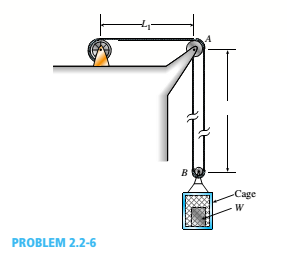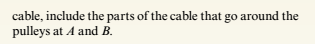Chapter 2, Problem 2.2.6P### Mechanics of Materials (MindTap Co...

9th Edition
Barry J. Goodno + 1 other
ISBN: 9781337093347
Textbook Problem

# By what distance h does the cage shown in the figure move downward when the weight W is placed inside it? (See the figure.)Consider only the effects of the stretching of the cable, which has axial rigidity EA = 10,700 kN. The pulley at A has a diameter da= 300 mm and the pulley at B has a diameter dB= 150 mm. Also, the distance L1= 4.6 m, the distance L2=10.5 m, and the weight W = 22 kN. Note: When calculating the length of the cable. include the parts of the cable that go around the pulley sat A and B.To determine

Distance h, when the weight W is placed inside the cage.

Explanation

Given information:

Axialrigidity(EA)=10700kNDiameterofpulleyatA(dA)=300mmDiameterofpulleyatB(dB)=150mmWeight(W)=22kNL1=4.6mL2=10.5m

Formula used:

Elongation(δ)=TLEA

Calculation:

Let us calculate the total length of cable;

L=L1+2L2+14(πdA)+12(πdB)</

### Still sussing out bartleby?

Check out a sample textbook solution.

See a sample solution

#### The Solution to Your Study Problems

Bartleby provides explanations to thousands of textbook problems written by our experts, many with advanced degrees!

Get Started

## Additional Engineering Solutions

#### Find more solutions based on key concepts